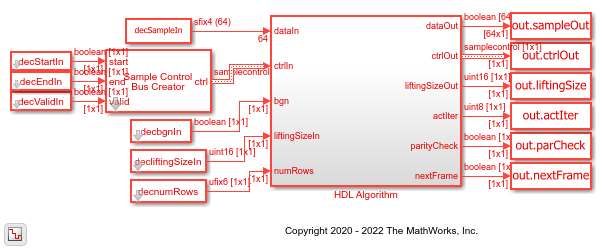# LDPC Decode 5G NR Streaming Data for Multiple Code Rates with Early Termination

This example shows how to use multiple code rates and early termination criteria features in the NR LDPC Decoder Simulink® block. The input to the block is generated using the `nrLDPCEncode` (5G Toolbox) MATLAB® function and the output of the block is compared with the input of the function. In this example, you can select either the min-sum or normalized min-sum algorithm for the decoding operation.

### Generate Input Data

Choose a series of input values for the base graph number (bgn) and liftingSize according to the 5G new radio (NR) standard and generate the corresponding input vectors for those values. Use the encoded data from the `nrLDPCEncode` function to generate input log-likelihood ratio (LLR) values for the NR LDPC Decoder block. Use channel, modulator, and demodulator System objects to add noise to the signal. Again, create vectors of `bgn` and `liftingSize`, and then convert the frames of data to LLRs with a control signal that indicates the frame boundaries. The `decFrameGap` accommodates the latency of the NR LDPC Decoder block for base graph number, liftingSize, and number of iterations. Use the nextFrame output signal to determine when the block is ready to accept the start of the next input frame.

```bgn = [1; 0; 0; 1]; liftingSize = [4; 384; 144; 208]; numRows = [6; 38; 24; 10]; numFrames = 4; serial = false; % true for serial inputs and false for parallel inputs msg = {numFrames}; K = []; N = []; for ii = 1:numFrames if bgn(ii) == 0 K(ii) = 22; else K(ii) = 10; end N(ii) = numRows(ii) + K(ii)-2; frameLen = liftingSize(ii)*K(ii); msg{ii} = randi([0 1],frameLen,1); encTmp = nrLDPCEncode(msg{ii},bgn(ii)+1); encOut{ii} = encTmp(1:N(ii)*liftingSize(ii)); end ```
```nVar = 0.5; chan = comm.AWGNChannel('NoiseMethod','Variance','Variance',nVar); bpskMod = comm.BPSKModulator; bpskDemod = comm.BPSKDemodulator('DecisionMethod', ... 'Approximate log-likelihood ratio','Variance',nVar); algo = 'Min-sum'; % 'Min-sum' or 'Normalized min-sum' if strcmpi(algo,'Min-sum') alpha = 1; else alpha = 0.75; end numIter = 8; decbgnIn = []; decliftingSizeIn = []; rxLLR = {numFrames}; decSampleIn = []; decStartIn = []; decEndIn = []; decValidIn = []; decnumRows = []; for ii=1:numFrames mod = bpskMod(double(encOut{ii})); rSig = chan(mod); rxLLR{ii} = fi(bpskDemod(rSig),1,4,0); if serial len = N(ii)*liftingSize(ii); %#ok<*UNRCH> decFrameGap = numIter*7000 + liftingSize(ii)*K(ii); else len = N(ii)*ceil(liftingSize(ii)/64); decFrameGap = numIter*1200; end decIn = ldpc_dataFormation(rxLLR{ii}',liftingSize(ii),N(ii),serial); decSampleIn = [decSampleIn decIn zeros(size(decIn,1),decFrameGap)]; %#ok<*AGROW> decStartIn = logical([decStartIn 1 zeros(1,len-1) zeros(1,decFrameGap)]); decEndIn = logical([decEndIn zeros(1,len-1) 1 zeros(1,decFrameGap)]); decValidIn = logical([decValidIn ones(1,len) zeros(1,decFrameGap)]); decbgnIn = logical([decbgnIn repmat(bgn(ii),1,len) zeros(1,decFrameGap)]); decliftingSizeIn = uint16([decliftingSizeIn repmat(liftingSize(ii),1,len) zeros(1,decFrameGap)]); decnumRows = fi([decnumRows repmat(numRows(ii),1,len) zeros(1,decFrameGap)],0,6,0); end decSampleIn = timeseries(fi(decSampleIn',1,4,0)); sampleTime = 1; simTime = length(decValidIn); ```

The `HDL Algorithm` subsystem contains the NR LDPC Decoder block. Running the model imports the input signal variables `decSampleIn`, `decStartIn`, `decEndIn`, `decValidIn`, `decbgnIn`, `decliftingSizeIn`, `numIter`, `sampleTime`, and `simTime` and exports a stream of decoded output samples `sampleOut` along with a control signal `ctrlOut` to the MATLAB workspace.

```open_system('NRLDPCDecoderCodeRateHDL'); if alpha ~= 1 set_param('NRLDPCDecoderCodeRateHDL/HDL Algorithm/NR LDPC Decoder','Algorithm','Normalized min-sum'); else set_param('NRLDPCDecoderCodeRateHDL/HDL Algorithm/NR LDPC Decoder','Algorithm','Min-sum'); end decOut = sim('NRLDPCDecoderCodeRateHDL'); ```### Compare Simulink Block Output with MATLAB Function Input

Convert the streaming data output of the NR LDPC Decoder block to frames and then compare the frames with the input of the `nrLDPCEncode` function.

```startIdx = find(decOut.ctrlOut.start.Data); endIdx = find(decOut.ctrlOut.end.Data); for ii = 1:numFrames decHDL{ii} = ldpc_dataExtraction(decOut.sampleOut.Data,liftingSize(ii),startIdx(ii),endIdx(ii),K(ii),serial); %#ok<*SAGROW> error = sum(abs(double(msg{ii})-decHDL{ii})); fprintf(['Decoded frame %d: Output data differs by %d bits\n'],ii,error); iter_tmp = squeeze(decOut.actIter.Data); actIter{ii} = iter_tmp(startIdx(ii)); fprintf(['Actual iterations taken to decode the frame: %d \n'],actIter{ii}); end ```
```Decoded frame 1: Output data differs by 0 bits Actual iterations taken to decode the frame: 2 Decoded frame 2: Output data differs by 0 bits Actual iterations taken to decode the frame: 2 Decoded frame 3: Output data differs by 0 bits Actual iterations taken to decode the frame: 2 Decoded frame 4: Output data differs by 0 bits Actual iterations taken to decode the frame: 3 ```